# Motion Speed Motion p Motion A change in

• Slides: 21Motion Speed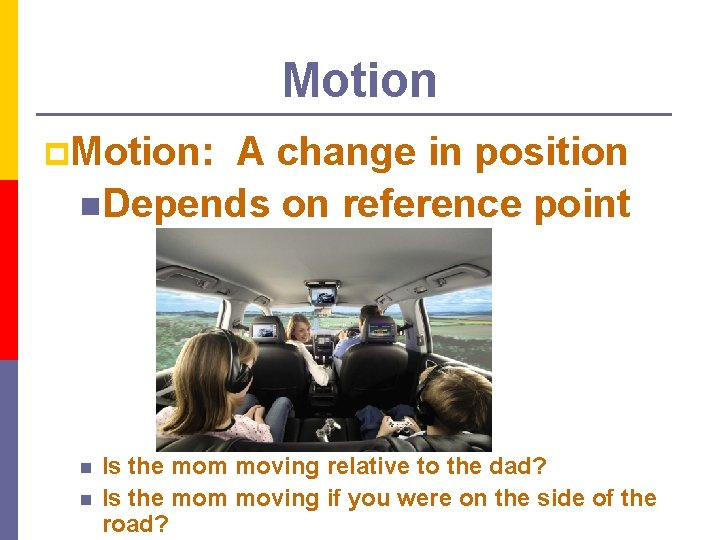Motion p. Motion: A change in position n. Depends on reference point n n Is the mom moving relative to the dad? Is the mom moving if you were on the side of the road?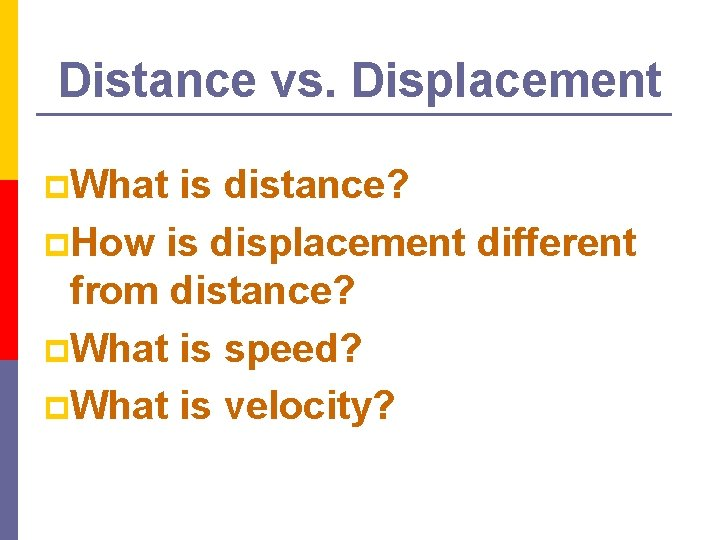Distance vs. Displacement p. What is distance? p. How is displacement different from distance? p. What is speed? p. What is velocity?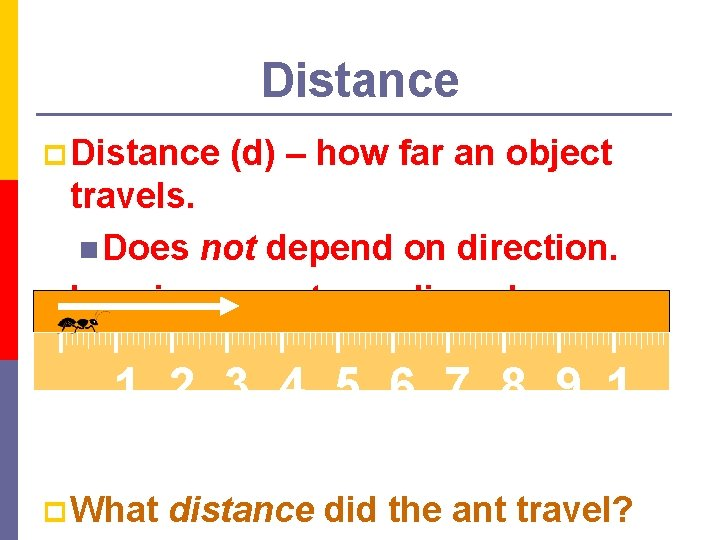Distance p Distance (d) – how far an object travels. n Does not depend on direction. p Imagine an ant crawling along a ruler. 1 2 3 4 5 6 7 8 9 1 0 p What distance did the ant travel?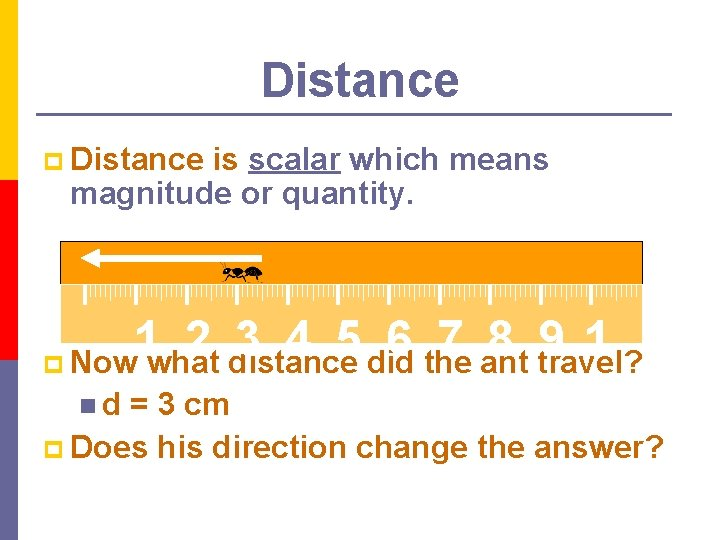Distance p Distance is scalar which means magnitude or quantity. 1 2 3 4 5 6 7 8 9 1 p Now what distance did the ant travel? 0 n d = 3 cm p Does his direction change the answer?Distance does not depend on direction. p Let’s follow the ant again. p 0 1 2 3 4 5 6 7 8 9 1 0 p What distance did the ant walk this time? p d = 7 cmDisplacement p Displacement ( x) – difference between an object’s final position and its starting position. n Does depend on direction. Displacement = final position – initial position p x = xfinal – xinitial p In order to define displacement, we need directions. p Examples of directions: p n n n + and – N, S, E, W AnglesDisplacement vs. Distance p. Example of distance: n. The ant walked 3 cm. p. Example of displacement: n. The ant walked 3 cm EAST. p. An object’s distance traveled and its displacement aren’t always the same!Displacement p Find the distance and displacement of the ant. - + 1 2 3 4 5 6 7 8 9 1 p Distance: 7 cm 0 p Displacement: +3 cm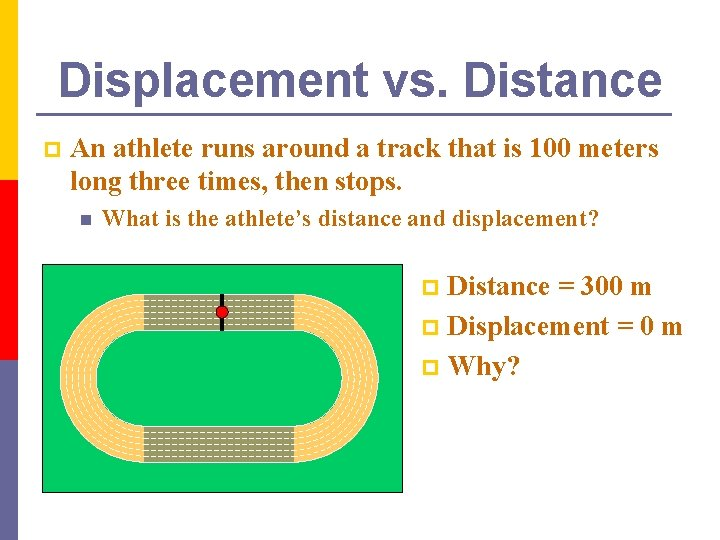Displacement vs. Distance p An athlete runs around a track that is 100 meters long three times, then stops. n What is the athlete’s distance and displacement? Distance = 300 m p Displacement = 0 m p Why? p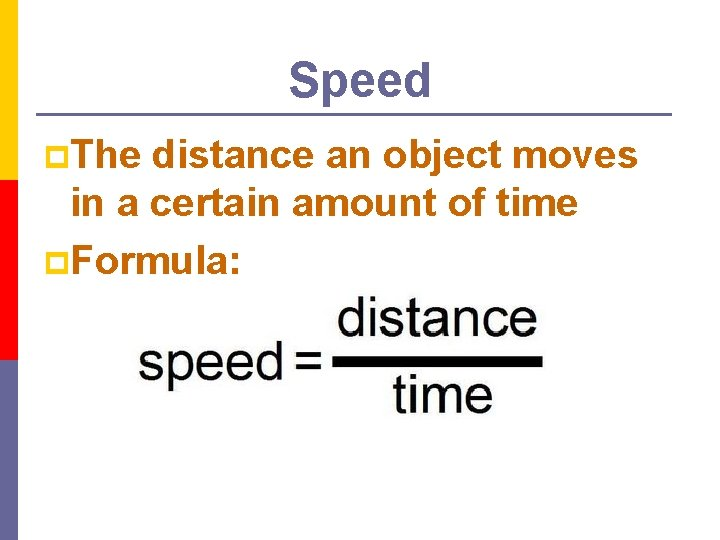Speed p. The distance an object moves in a certain amount of time p. Formula:Unit for Speed p Since p Then the formula is the unit for speed must be a unit of distance divided by a unit of time. p For example: n km/h n m/sSpeed p. Look familiar? p. Knowing any of the 2 variables, you can solve for the 3 rd.Calculating Speed p. A car drives 100 meters in 5 seconds. p What is the car’s average speed? n s = d/t n s = (100 m) / (5 s) = 20 m/s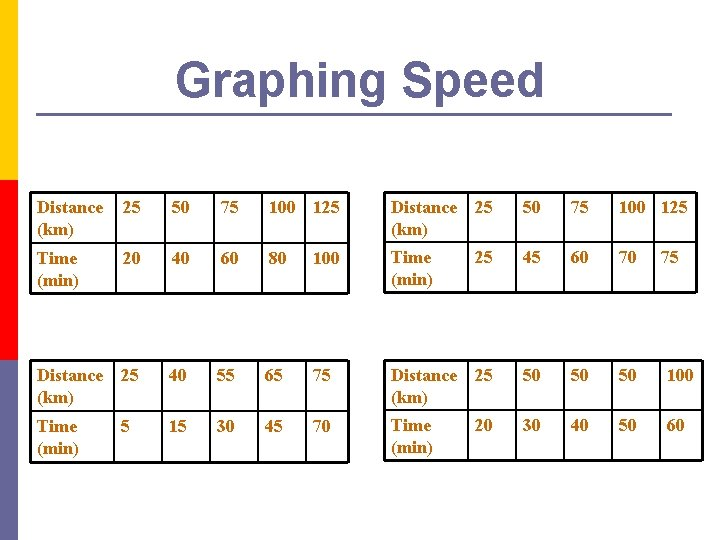Graphing Speed Distance (km) 25 50 75 100 125 Time (min) 20 40 60 80 100 Time (min) 25 45 60 70 Distance (km) 25 40 55 65 75 Distance (km) 25 50 50 50 100 Time (min) 5 15 30 45 70 Time (min) 20 30 40 50 60 75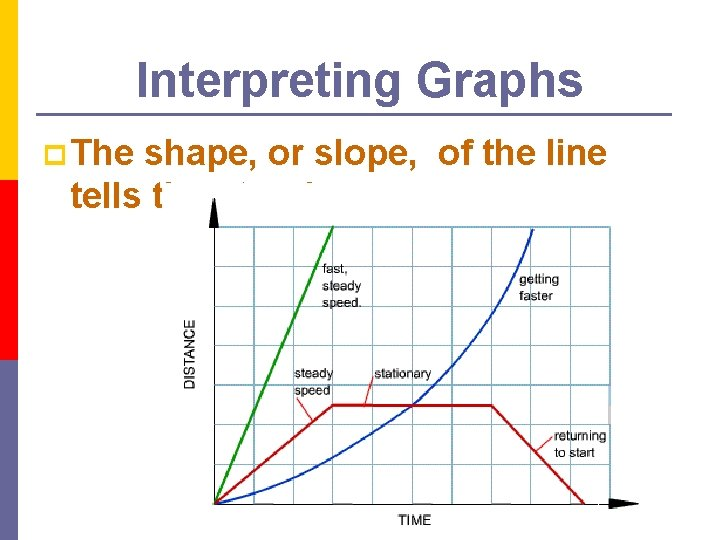Interpreting Graphs p The shape, or slope, of the line tells the story!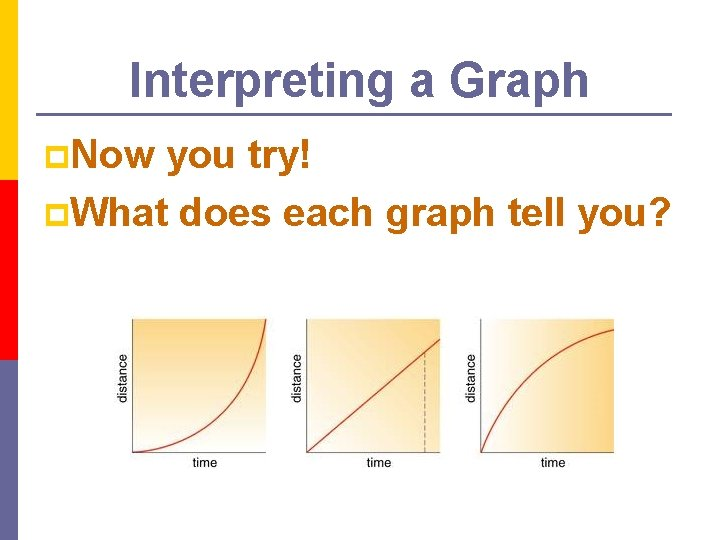Interpreting a Graph p. Now you try! p. What does each graph tell you?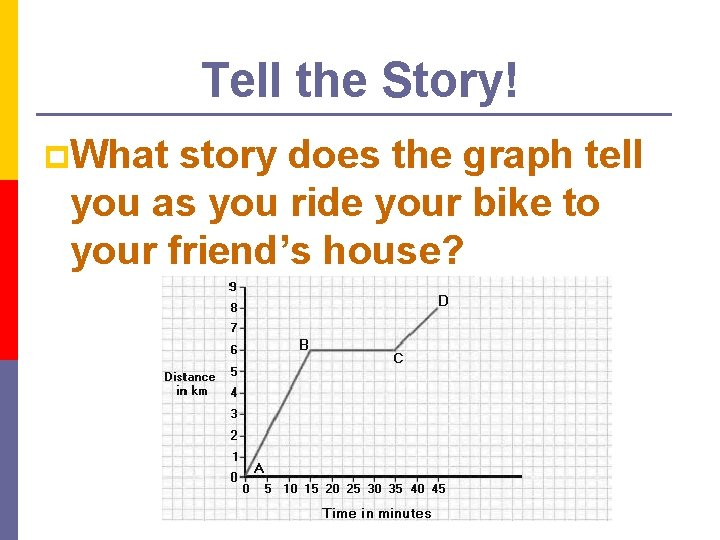Tell the Story! p. What story does the graph tell you as you ride your bike to your friend’s house?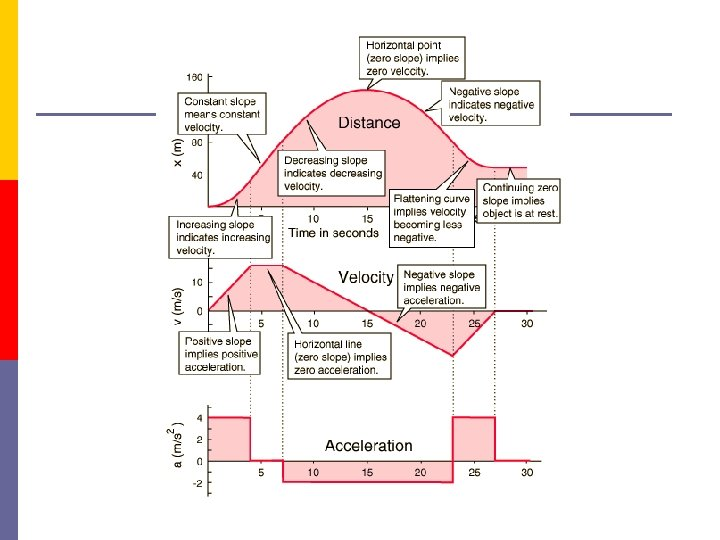Interpret This GraphScalar vs. Vector p Scalar Quantity – has magnitude but not direction. n Distance and speed are scalar quantities. p Vector Quantity – has magnitude and direction. n Displacement and velocity are vector quantities.